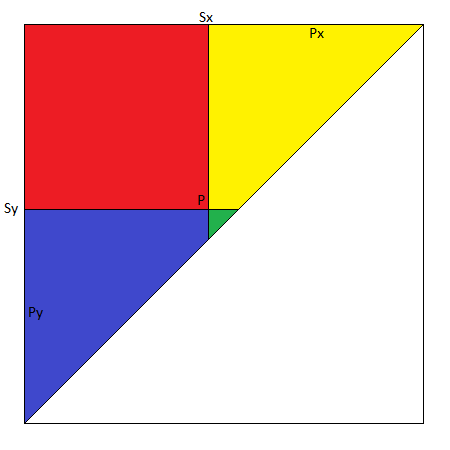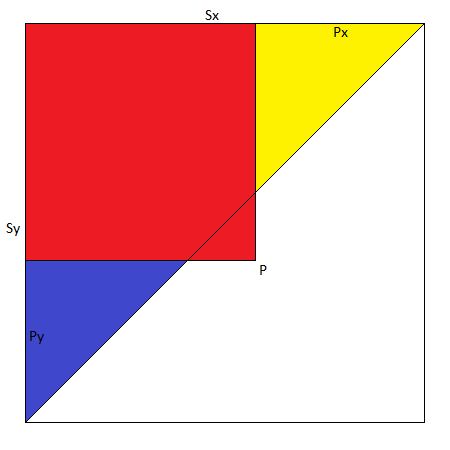While playing around with XNA and my simple Snooker game I bumped into a geometrical problem, basically I wanted to know if the ball coordinate is in the corner of the table, which in my simple game equates to determining if a coordinate is inside a triangle near table corner.

I was looking at the algorithm I wrote in the original DirectX version of this game and quite frankly I had no idea what I was doing there. Speaking of which, my code from 10 years ago is quite shocking, I came a long way since :)

Anyway, after scratching my bald head for a while I came up with this.

I am dealing with a triangle that has two sides of the same length, mathematicians would call it an Isosceles triangle. What I did first was calculate how much into the pocket the ball was along X and Y axises and if the sum of these two is bigger than the side of the triangle then I assume the coordinate is inside triangle.

In the image below Px + Py is greater than Sx (which is the same as Sy since the sides are of equal length) and P is inside triangle.Now consider the image below, here the sum of Px and Py is less than Sx (Sy) and my coordinate is outside of the triangle.